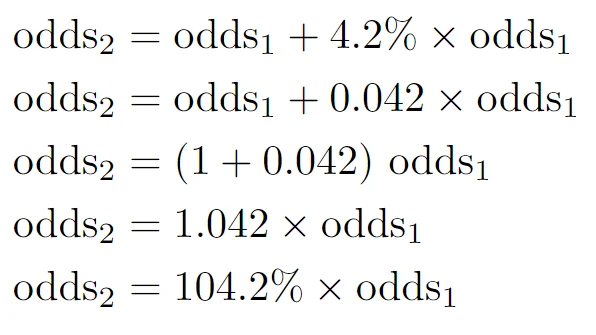29 Aug 2022

Posted on:

29 Aug 2022

0

# Trouble understanding the last step in the coefficient interpretation

At 3:23, how did we go from odds2=104.2%*odds1, to "when the SAT score increases by 1, the odds of admittance increase by 4.2%"?

Posted on:

29 Aug 2022

0

Hey Kareem,

Thank you for reaching out!

Let's work with the following sentence:
'odds2 is 4.2% higher than odds1.'
How would one go about calculating odds2? We would need to find 4.2% of odds1 and add that to the value of odds1, namely:We have therefore arrived at the same expression as the one in the lecture. Increasing odds1 by 4.2% is equivalent to multiplying odds1 by 100%+4.2% = 104.2%.

Analogously, decreasing a number by 4.2% would be equivalent to multiplying the number by 100%-4.2% = 95.8%. It's simply a more straightforward way of increasing or decreasing a number by a given percent.

Hope this helps!

Kind regards,
365 Hristina